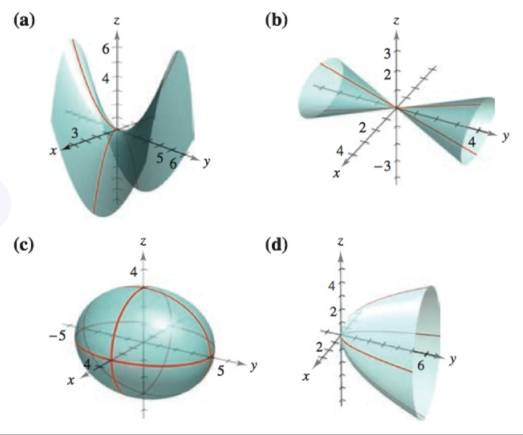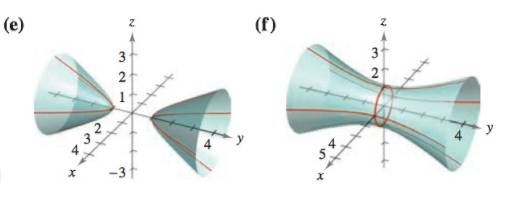Chapter 11.6, Problem 6E

Chapter
Section
Textbook Problem

# Matching In Exercises 5-10, match the equation with its graph. [The graphs are labeled (a), (b), (c), (d), (e), and (F).]4 x 2 − y 2 + 4 z = 0

To determine

To calculate: Match the correct option of the equation 4x2y2+4z=0.

Explanation

Given:

The provided equation is:

4x2y2+4z=0

The provided graph are:

Calculation:

Consider the table of the quadric surfaces with their standard equation is shown below,

The equation is,

4x2y2+4z=0

Simplify the above equation,

x21y24+z1=0

The xy- trace (z=1) is,

x21y24=1y24x21=1

Trace the above equation that represent a hyperbola

### Still sussing out bartleby?

Check out a sample textbook solution.

See a sample solution

#### The Solution to Your Study Problems

Bartleby provides explanations to thousands of textbook problems written by our experts, many with advanced degrees!

Get Started

#### In problems 49-54, rationalize the denominator and then simpify. 51.

Mathematical Applications for the Management, Life, and Social Sciences

#### Evaluate the integral. 19. x+19x2+6x+5dx

Single Variable Calculus: Early Transcendentals

#### True or False: n=1(1)nn+14 is a convergent series.

Study Guide for Stewart's Single Variable Calculus: Early Transcendentals, 8th

#### For

Study Guide for Stewart's Multivariable Calculus, 8th

#### Graph each function. fx=-x2-6x-5

College Algebra (MindTap Course List)